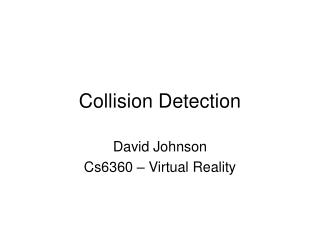DownloadDownload PresentationCollision Detection

# Collision Detection

Download Presentation## Collision Detection

- - - - - - - - - - - - - - - - - - - - - - - - - - - E N D - - - - - - - - - - - - - - - - - - - - - - - - - - -
##### Presentation Transcript

1. Collision Detection David Johnson Cs6360 – Virtual Reality

2. Basic Problems • Too many objects • Discrete Sampling • Complex Objects

3. Too Many Objects • Even if cheap to detect collisions, will have n^2 checks • http://www.youtube.com/watch?v=OBzBfhrjx5s&feature=related

4. Tunneling

5. Tunneling

6. Tunneling

7. Tunneling

8. Complex Objects • N^2 problem between triangles of individual models

9. Approaches • Too many objects • Partition Space • Velocity Bounds

10. Spatial Partition • Uniform Grid

11. Interval Projection • Project bounding volume on each axis • Maintain sorted lists • Possible collision when intervals overlap

12. Tunneling • Fundamentally a sampling problem • If you know the max velocity and minimum thickness, can bound the error

13. Tunneling • Fundamentally a sampling problem • If you know the max velocity and minimum thickness, can bound the error

14. Sweep Methods • Sweep out the volume along the path • Different accuracy choices • Test for collisions • False positives • Bisect the interval • Rotations are tough

15. Time of collision • Interval Halving • Conservative Advancement • Minkowski Difference/ray-cast

16. Collision for Complex Models • Models may have millions of primitives • Not moving independently, so sweep methods are overkill • Spatial partitions are tough to update • May be in close proximity for extended periods • Need to avoid false positives

17. Bounding Volumes • Objects are often not colliding • Need fast reject for this case • Surround with some bounding object • Like a sphere • Why stop with one layer of rejection testing? • Build a bounding volume hierarchy (BVH) • A tree

18. Bounding Volume Hierarchies • Model Hierarchy: • each node has a simple volume that bounds a set of triangles • children contain volumes that each bound a different portion of the parent’s triangles • The leaves of the hierarchy usually contain individual triangles • A binary bounding volume hierarchy:

19. BVH-Based Collision Detection

20. Type of Bounding Volumes • Spheres • Ellipsoids • Axis-Aligned Bounding Boxes (AABB) • Oriented Bounding Boxes (OBBs) • Convex Hulls • k-Discrete Orientation Polytopes (k-dop) • Spherical Shells • Swept-Sphere Volumes (SSVs) • Point Swept Spheres (PSS) • Line Swept Spheres (LSS) • Rectangle Swept Spheres (RSS) • Triangle Swept Spheres (TSS)

21. BV Choices • OBB or AABB • OBB slightly better for close proximity • AABB better for handling deformations

22. Recursive top-down construction: partition and refit Building an OBBTree

23. Given some polygons, consider their vertices... Building an OBB Tree

24. … and an arbitrary line Building an OBB Tree

25. Building an OBB Tree Project onto the line Consider variance of distribution on the line

26. Building an OBB Tree Different line, different variance

27. Maximum Variance Building an OBB Tree

28. Minimal Variance Building an OBB Tree

29. Given by eigenvectors of covariance matrix of coordinates of original points Building an OBB Tree

30. Choose bounding box oriented this way Building an OBB Tree

31. Good Box Building an OBB Tree

32. Add points: worse Box Building an OBB Tree

33. More points: terrible box Building an OBB Tree

34. Compute with extremal points only Building an OBB Tree

35. Building an OBB Tree “Even” distribution: good box

36. “Uneven” distribution: bad box Building an OBB Tree

37. Fix: Compute facets of convex hull... Building an OBB Tree

38. Better: Integrate over facets Building an OBB Tree

39. … and sample them uniformly Building an OBB Tree

40. Tree Traversal Disjoint bounding volumes: No possible collision

41. Tree Traversal • Overlapping bounding volumes: • split one box into children • test children against other box

42. Tree Traversal

43. Tree Traversal Hierarchy of tests

44. SeparatingAxis Theorem • L is a separating axis for OBBs A & B, since A & B become disjoint intervals under projection onto L

45. Separating Axis Theorem Two polytopes A and B are disjoint iff there exists a separating axis which is: perpendicular to a face from either or perpendicular to an edge from each

46. Implications of Theorem Given two generic polytopes, each with E edges and F faces, number of candidate axes to test is: 2F + E2 OBBs have only E = 3 distinct edge directions, and only F = 3 distinct face normals. OBBs need at most 15 axis tests. Because edge directions and normals each form orthogonal frames, the axis tests are rather simple.

47. h h s > + L is a separating axis iff: OBB Overlap Test: An Axis Test L s h a h b b a

48. OBB Overlap Test: Axis Test Details Box centers project to interval midpoints, so midpoint separation is length of vector T’s image.

49. OBB Overlap Test: Axis Test Details • Half-length of interval is sum of box axis images.

50. OBB Overlap Test • Strengths of this overlap test: • 89 to 252 arithmetic operations per box overlap test • Simple guard against arithmetic error • No special cases for parallel/coincident faces, edges, or vertices • No special cases for degenerate boxes • No conditioning problems • Good candidate for micro-coding